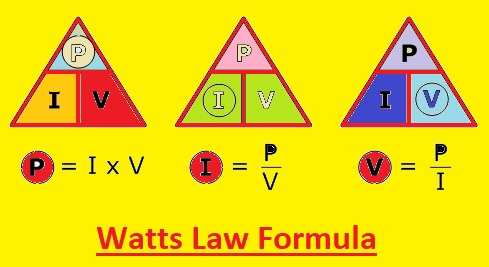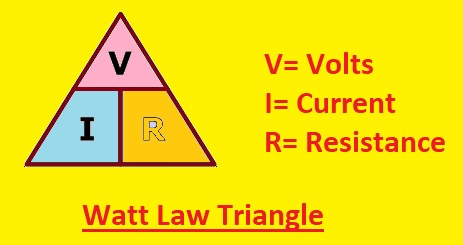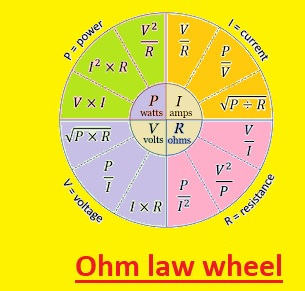Hello, readers welcome to the new post. In this post, we will discuss Introduction to Watts Law. What’s law explains the relationship between voltage current and power and defines that power in circuitry is multiple of voltage and current. The normal formula used for measurement of watt’s law is P=VI since it found power so this formula is like the power equation. Through using this law we can find the current volts, power, and resistance of any circuitry.

Through making combinations with Ohm’s law we can get a different expression that will help us to find different values in circuits. For solutions of different circuits, there are different laws are applied that help us to find different parameters of circuit components. Like these laws watt’s law is used to find power volts and current. In this post, we will discuss different parameters to find the Watt law. So let’s get started with Introduction to Watts Law

## Table of Content:

What is Watts Law?

Watts Law Formula

Watts Law Examples

Watts Law Vs. Ohms Law

Watts Law Triangle

### Watts Law parameters

Applications of Watts law

## What is Watts Law?

• Watt’s law makes the relation between power, ampere, and voltage in the circuitry of different components. It states that voltage and current multiple are equal to power.

## Watts Law Formula (edited)

• The formula of Watts Law is given here
• Power= Voltage x Current or P=V/I
• Voltage =Power/Current or V=P/I
• Current= Power/ Voltage I=P/V### Watts Law Examples

• Here are some examples of Watts law solved
• The one hundred-watt light bulb is attached to 120 volts power supply. Through the use of Watts Law, we can find the current flowing through the light bulb
• P = I * V
• 100 = I * 120
• I = 0.83 A
• The current flowing in the bulb will be  0.83 A.
• The hairdryer uses 1200 watts of power when having a connection with a 120-volt power supply. Here we will apply the watts law and finds the current flowing in the hairdryer P = I x V
• 1200= I x 120
• I = 10 A
• Here 10 amperes current flowing in the dryer.

## Watts Law Vs. Ohms Law

• Watts’s law and Ohm’s law are two basic principles that discuss the behavior of current in circuits. Watts law defines the relationship between current volts and power in circuits. Ohm’s law explains and defines the voltage, current, and resistance relationship. These two laws are interrelated and can be used for the solution of different electrical circuits
• According to Ohm’s law current flowing through the circuit is directly proportional to the voltage applied and inverse relation with the resistance of the circuitry. The formula of Ohms law is

I = V / R

• Here the current is I, R is resistance, and  is the voltage
• If we put Ohms’ law in Watts’s law then we can new relationship

P = V^2 / R or P = I^2 x R

• The above expression explains that power used by the electrical device is directly proportional to the voltage applied to square and inversely proportional to circuit resistance
Watts Law Ohm’s Law
It measures power consumption in a circuit It finds values of  current and resistance in a circuit
Formula is P = V^2 / R or P = I^2 x R Its  Formula: I = V / R
It defines the relationship between power, voltage, and resistance It makes the relationship between voltage, current, and resistance
Apply for calculation of  electrical components for a circuit it is applied for determining the resistance of a circuit
Assumes ideal components with no inner resistance or losses Takes into account the circuit resistance

## Watts Law Triangle

• Watts law triangle is a graphical representation of the P = V x I formula that makes the relationship between power voltage and current in circuits
• This triangle is created through three lines drawing with P exiting at the upper point, V, and I at the bottom point.
• For use triangle just use the variable that you have to find and the formula will be configured according to that.
• For example for calculation of power of 100 watts in circuitry and current of five amperes. We will arrange the formula as V = P / I, so V = 100 / 5 = 20 V.
• The watts law triangle is beneficial for the calculation of different parameters like power volts and current of circuits### Ohms Law Wheel

• The Ohm’s law wheel is circle shape diagram that indicates the relationship between current volts and resistance R and power in the circuit.
• It is normally used tool in circuits for the calculation of circuits parameters
• There are four parts of the wheel and each denoting four variables arrows indicating the direction of these parameters
• Let’s suppose the values of voltage and current we know in circuitry then through the use of a wheel we can find resistance or power
• To use the wheel just rotate two known parameters or variables till they are aligned and the values of two variables can be discussed on the basis of intersecting lines
• The Ohm’s law wheel is beneficial for electric circuits engineers and power system analysis### Watts Law Parameters

• The main parameters of the watts law are voltage, current, and resistance

Voltage

• Voltage is the potential difference between 2 points of circuitry. The difference in potential results in the flow of electrons from high potential to low potential. The unit of volts is the voltage

Current

• Current is the flow of charges and their units are amperes. Current only flow when there is a potential difference exists between two points.

Resistance

• The opposition in the flow of current is called resistance and its measuring unit is the ohm

Power

• Power is a measurement of the amount of work done in circuitry or power used by components in unit time. In a simple way, power is the amount of energy transferred in unit time. The unit of power is watt or J//s

## Applications of Watts law

• Through the use of power sources, we can find valuable factual power sources generated by the source. We can use it to find the power used by the component in circuits. To find the power current and voltage source multiply them
• Power needs can be calculated through the use of the watt formula. During the wiring design creation, it is compulsory to have an estimation of electricity charges. The power need of creation is done by measuring the power rating of each device.
• If we have a value of power and volts of the component we can find the current through the use of the watt formula I=P/V. The same is also done for voltage when current and power value is given
• The equation found from Watt and Ohm law can be applied to find the resistance of the component.

FAQs:

### What are Ohm’s law and Watts’s law?

These two laws are fundamental laws of the electrical system. Ohm’s law describes current flowing in conductors among two points as directly proportional to the voltage applied. Watts law,  states that power used by a circuit depends on the voltage and current of the circuitry

### What is the unit of watts law?

The unit of watts law is watts that denotes power consumed by the circuit

### What is the power law in electricity?

The power law of electricity defines the relation between power volts and the current of circuitry. It defines that power used in a circuit equal to voltage and current multiple

### Why is it called power law?

It is known as power law since it makes define the power used by the circuit. Power is the rate of energy transferred and the power used by the circuit is equal to the multiple of voltage and current

### What is the power law formula?

The formula of the power law is P= V* I

P is power, V is volts and I is current………….